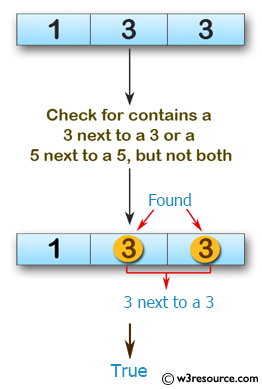﻿ Swift Array Programming Exercise: Check if a given array of integers contains a 3 next to a 3 or a 5 next to a 5, but not both - w3resource# Swift Array Programming Exercises: Check if a given array of integers contains a 3 next to a 3 or a 5 next to a 5, but not both

## Swift Array Programming: Exercise-31 with Solution

Write a Swift program to check if a given array of integers contains a 3 next to a 3 or a 5 next to a 5, but not both.

Pictorial Presentation:Sample Solution:

Swift Code:

``````func num35(array_nums: [Int]) -> Bool{
var num_3 = false
var num_5 = false

for x in 0..<array_nums.count-1 {
if array_nums[x] == 3 && array_nums[x+1] == 3
{
num_3 = true
}
else if array_nums[x] == 5 && array_nums[x+1] == 5
{
num_5 = true
}
}

return (num_3 && !num_5) || (!num_3 && num_5)
}

print(num35(array_nums: [1, 3, 3]))
print(num35(array_nums: [5, 5, 1]))
print(num35(array_nums: [5, 5, 1, 3, 3]))
```
```

Sample Output:

```true
true
false
```

Swift Programming Code Editor:

Improve this sample solution and post your code through Disqus

What is the difficulty level of this exercise?

﻿